Search by Topic

Resources tagged with Digit cards similar to Twenty Divided Into Six:

Filter by: Content type:
Age range:
Challenge level:

There are 12 results

Broad Topics > Mathematics Tools > Digit cards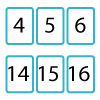Twenty Divided Into Six

Age 7 to 11 Challenge Level:

Katie had a pack of 20 cards numbered from 1 to 20. She arranged the cards into 6 unequal piles where each pile added to the same total. What was the total and how could this be done?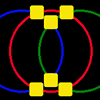Magic Circles

Age 7 to 11 Challenge Level:

Put the numbers 1, 2, 3, 4, 5, 6 into the squares so that the numbers on each circle add up to the same amount. Can you find the rule for giving another set of six numbers?Sealed Solution

Age 7 to 11 Challenge Level:

Ten cards are put into five envelopes so that there are two cards in each envelope. The sum of the numbers inside it is written on each envelope. What numbers could be inside the envelopes?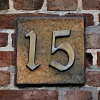Finding Fifteen

Age 7 to 11 Challenge Level:

Tim had nine cards each with a different number from 1 to 9 on it. How could he have put them into three piles so that the total in each pile was 15?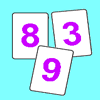Penta Primes

Age 7 to 11 Challenge Level:

Using all ten cards from 0 to 9, rearrange them to make five prime numbers. Can you find any other ways of doing it?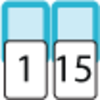Fifteen Cards

Age 7 to 11 Challenge Level:

Can you use the information to find out which cards I have used?All the Digits

Age 7 to 11 Challenge Level:

This multiplication uses each of the digits 0 - 9 once and once only. Using the information given, can you replace the stars in the calculation with figures?Magic Vs

Age 7 to 11 Challenge Level:

Can you put the numbers 1-5 in the V shape so that both 'arms' have the same total?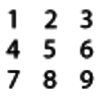Four-digit Targets

Age 7 to 11 Challenge Level:

You have two sets of the digits 0 – 9. Can you arrange these in the five boxes to make four-digit numbers as close to the target numbers as possible?The Thousands Game

Age 7 to 11 Challenge Level:

Each child in Class 3 took four numbers out of the bag. Who had made the highest even number?Song Book

Age 7 to 11 Challenge Level:

A school song book contains 700 songs. The numbers of the songs are displayed by combining special small single-digit cards. What is the minimum number of small cards that is needed?Reach 100

Age 7 to 14 Challenge Level:

Choose four different digits from 1-9 and put one in each box so that the resulting four two-digit numbers add to a total of 100.